## Loren on the Art of MATLABTurn ideas into MATLAB

Note

Loren on the Art of MATLAB has been retired and will not be updated.

# A Mandelbrot Set on the GPU

Today I welcome back guest blogger Ben Tordoff who last blogged here on how to Carve a Dinosaur. These days Ben works on the Parallel Computing Toolbox™ with particular focus on GPUs.

### Contents

Benoit Mandelbrot died last Autumn, and despite inventing the term "fractal" and being one of the pioneers of fractal geometry, his lasting legacy for most people will undoubtedly be the fractal that bears his name. There can be few mathematicians or mathematical algorithms that have adorned as many teenage bedroom walls as Mandelbrot's set did in the late '80s and early '90s.

I also suspect there is no other fractal that has been implemented in quite as many different languages on different platforms. My first version was a blocky 32x24 version on an aging ZX Spectrum with seven glorious colours. These days we have a bit more graphical power available, and a few more colours. In fact, as we shall see, an algorithm like the Mandelbrot Set is ideally suited to running on that GPU (Graphics Processing Unit) which mostly sits idle in your PC.

As a starting point I will use a version of the Mandelbrot set taken loosely from Cleve Moler's Experiments with MATLAB e-book. We will then look at the three different ways that the Parallel Computing Toolbox™ provides for speeding this up using the GPU:

1. Using the existing algorithm but with GPU data as input
2. Using arrayfun to perform the algorithm on each element independently
3. Using the MATLAB/CUDA interface to run some existing CUDA/C++ code

As you will see, these three methods are of increasing difficulty but repay the effort with hugely increased speed. Choosing a method to trade off difficulty and speed is typical of parallel problems.

### Setup

The values below specify a highly zoomed part of the Mandelbrot Set in the valley between the main cardioid and the p/q bulb to its left.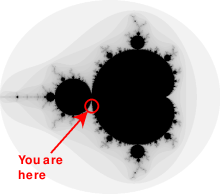The following code forms the set of starting points for each of the calculations by creating a 1000x1000 grid of real parts (X) and imaginary parts (Y) between these limits. For this particular location I happen to know that 500 iterations will be enough to draw a nice picture.

maxIterations = 500;
gridSize = 1000;
xlim = [-0.748766713922161, -0.748766707771757];
ylim = [ 0.123640844894862,  0.123640851045266];

### The Mandelbrot Set in MATLAB

Below is an implementation of the Mandelbrot Set using standard MATLAB commands running on the CPU. This calculation is vectorized such that every location is updated at once.

% Setup
t = tic();
x = linspace( xlim(1), xlim(2), gridSize );
y = linspace( ylim(1), ylim(2), gridSize );
[xGrid,yGrid] = meshgrid( x, y );
z0 = xGrid + 1i*yGrid;
count = zeros( size(z0) );

% Calculate
z = z0;
for n = 0:maxIterations
z = z.*z + z0;
inside = abs( z )<=2;
count = count + inside;
end
count = log( count+1 );

% Show
cpuTime = toc( t );
set( gcf, 'Position', [200 200 600 600] );
imagesc( x, y, count );
axis image
colormap( [jet();flipud( jet() );0 0 0] );
title( sprintf( '%1.2fsecs (without GPU)', cpuTime ) );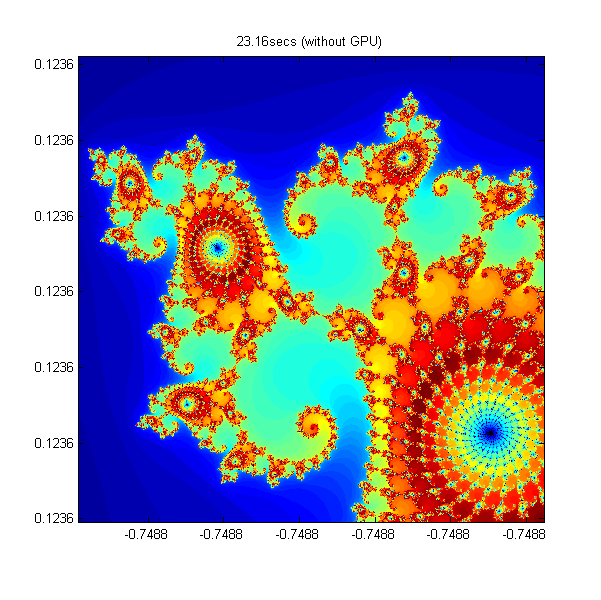### Using parallel.gpu.GPUArray - 16 Times Faster

parallel.gpu.GPUArray provides GPU versions of many functions that can be used to create data arrays, including the linspace, logspace, and meshgrid functions needed here. Similarly, the count array is initialized directly on the GPU using the function parallel.gpu.GPUArray.zeros.

Other than these simple changes to the data initialization, the algorithm is unchanged (and >16 times faster):

% Setup
t = tic();
x = parallel.gpu.GPUArray.linspace( xlim(1), xlim(2), gridSize );
y = parallel.gpu.GPUArray.linspace( ylim(1), ylim(2), gridSize );
[xGrid,yGrid] = meshgrid( x, y );
z0 = complex( xGrid, yGrid );
count = parallel.gpu.GPUArray.zeros( size(z0) );

% Calculate
z = z0;
for n = 0:maxIterations
z = z.*z + z0;
inside = abs( z )<=2;
count = count + inside;
end
count = log( count+1 );

% Show
naiveGPUTime = toc( t );
imagesc( x, y, count )
axis image
title( sprintf( '%1.2fsecs (naive GPU) = %1.1fx faster', ...
naiveGPUTime, cpuTime/naiveGPUTime ) )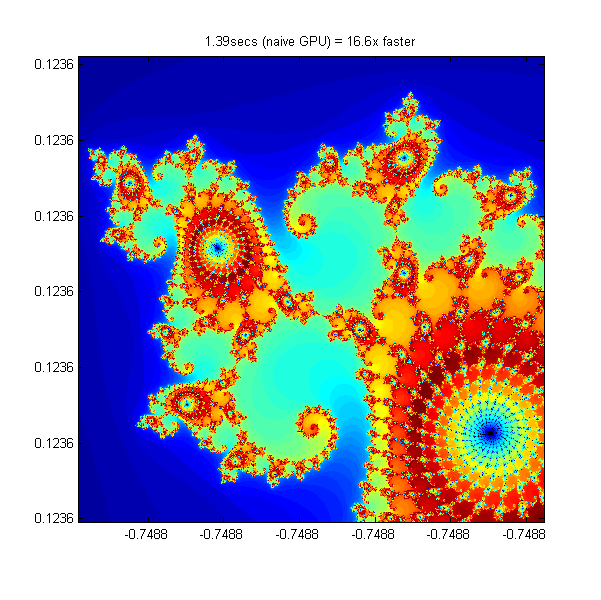### Using Element-wise Operation - 164 Times Faster

Noticing that the algorithm is operating equally on every element of the input, we can place the code in a helper function and call it using arrayfun. For GPU array inputs, the function used with arrayfun gets compiled into native GPU code. In this case we placed the loop in processMandelbrotElement.m which looks as follows:

function count = processMandelbrotElement(x0,y0,maxIterations)
z0 = complex(x0,y0);
z = z0;
count = 1;
while (count <= maxIterations) ...
&& ((real(z)*real(z) + imag(z)*imag(z)) <= 4)
count = count + 1;
z = z*z + z0;
end
count = log(count);

Note that an early abort has been introduced since this function only processes a single element. For most views of the Mandelbrot Set a significant number of elements stop very early and this can save a lot of processing. The for loop has also been replaced by a while loop because they are usually more efficient. This function makes no mention of the GPU and uses no GPU-specific features - it is standard MATLAB code.

Using arrayfun means that instead of many thousands of calls to separate GPU-optimized operations (at least 6 per iteration), we make one call to a parallelized GPU operation that performs the whole calculation. This significantly reduces overhead - 164 times faster.

% Setup
t = tic();
x = parallel.gpu.GPUArray.linspace( xlim(1), xlim(2), gridSize );
y = parallel.gpu.GPUArray.linspace( ylim(1), ylim(2), gridSize );
[xGrid,yGrid] = meshgrid( x, y );

% Calculate
count = arrayfun( @processMandelbrotElement, xGrid, yGrid, maxIterations );

% Show
gpuArrayfunTime = toc( t );
imagesc( x, y, count )
axis image
title( sprintf( '%1.2fsecs (GPU arrayfun) = %1.1fx faster', ...
gpuArrayfunTime, cpuTime/gpuArrayfunTime ) );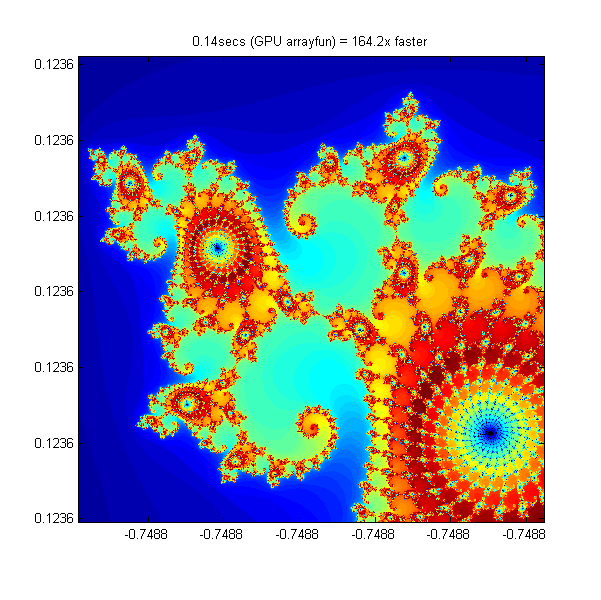### Working in CUDA - 340 Times Faster

In Experiments in MATLAB improved performance is achieved by converting the basic algorithm to a C-Mex function. If you're willing to do some work in C/C++, then you can use the Parallel Computing Toolbox to call pre-written CUDA kernels using MATLAB data. This is done using the toolbox's parallel.gpu.CUDAKernel feature.

A CUDA/C++ implementation of the element processing algorithm has been hand-written in processMandelbrotElement.cu. This must then be manually compiled using nVidia's NVCC compiler to produce the assembly-level processMandelbrotElement.ptx (.ptx stands for "Parallel Thread eXecution language").

The CUDA/C++ code is a little more involved than the MATLAB versions we've seen so far due to the lack of complex numbers in C++. However, the essence of the algorithm is unchanged:

__device__
unsigned int doIterations( double const realPart0,
double const imagPart0,
unsigned int const maxIters ) {
// Initialize: z = z0
double realPart = realPart0;
double imagPart = imagPart0;
unsigned int count = 0;
// Loop until escape
while ( ( count <= maxIters )
&& ((realPart*realPart + imagPart*imagPart) <= 4.0) ) {
++count;
// Update: z = z*z + z0;
double const oldRealPart = realPart;
realPart = realPart*realPart - imagPart*imagPart + realPart0;
imagPart = 2.0*oldRealPart*imagPart + imagPart0;
}
return count;
}

(the full source-code is available in the file-exchange submission linked at the end.)

One GPU thread is required for each element in the array, divided into blocks. The kernel indicates how big a thread-block is, and this is used to calculate the number of blocks required.

% Setup
t = tic();
x = parallel.gpu.GPUArray.linspace( xlim(1), xlim(2), gridSize );
y = parallel.gpu.GPUArray.linspace( ylim(1), ylim(2), gridSize );
[xGrid,yGrid] = meshgrid( x, y );

kernel = parallel.gpu.CUDAKernel( 'processMandelbrotElement.ptx', ...
'processMandelbrotElement.cu' );

% Make sure we have sufficient blocks to cover the whole array
numElements = numel( xGrid );

% Call the kernel
count = parallel.gpu.GPUArray.zeros( size(xGrid) );
count = feval( kernel, count, xGrid, yGrid, maxIterations, numElements );

% Show
gpuCUDAKernelTime = toc( t );
imagesc( x, y, count )
axis image
title( sprintf( '%1.2fsecs (GPU CUDAKernel) = %1.1fx faster', ...
gpuCUDAKernelTime, cpuTime/gpuCUDAKernelTime ) );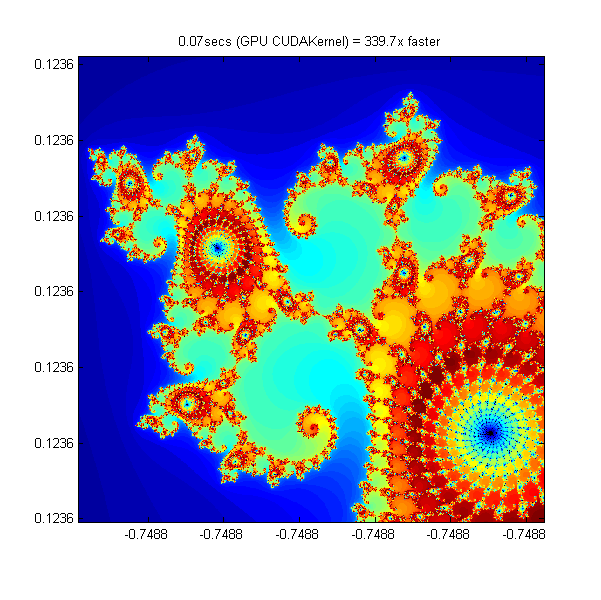### Summary

When my brothers and I sat down and coded up our first Mandelbrot set it took over a minute to render a 32x24 image. Here we have seen how some simple steps and some nice hardware can now render 1000x1000 images at several frames per second. How times change!

We have looked at three ways of speeding up the basic MATLAB implementation:

1. Convert the input data to be on the GPU using gpuArray, leaving the algorithm unchanged
2. Use arrayfun on a GPUArray input to perform the algorithm on each element of the input independently
3. Use parallel.gpu.CUDAKernel to run some existing CUDA/C++ code using MATLAB data

I've also created a graphical application that lets you explore the Mandelbrot Set using each of these approaches. You can download this from the File Exchange:

If you have any ideas for creating Mandelbrot Sets at even greater speed or can think of other algorithms that might make good use of the GPU, please leave your comments and questions below.

### References

Read a bit more about the life and times of Benoit Mandelbrot:

See his recent talk at TED:

Have a look at Cleve Moler's chapter on the Mandelbrot Set: